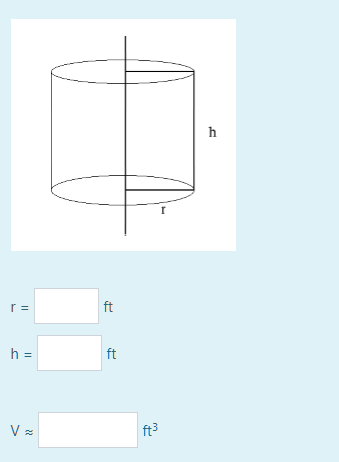Answer should be in decimal form. Up to two decimal places only

reopened

 Your name to display (optional): Email me at this address if my answer is selected or commented on: Privacy: Your email address will only be used for sending these notifications. Anti-spam verification: To avoid this verification in future, please log in or register.

The perimeter of the rectangle is 2(r+h)=12, so h=6-r.

Volume of cylinder is V=πr²(6-r)=6πr²-πr³, dV/dr=12πr-3πr²=0 at max or min. Divide through by 3π:

4r-r²=0=r(4-r), so r=0 is the minimum, and r=4 inches, therefore h=2 inches.

The unit of measurement in the question is inches, but the answer is expected to be in feet, so r=4/12=0.33ft and h=2/12=0.17ft. V=32π cubic inches=100.53 cubic inches or 0.06 cu ft.

by Top Rated User (1.0m points)
selected by